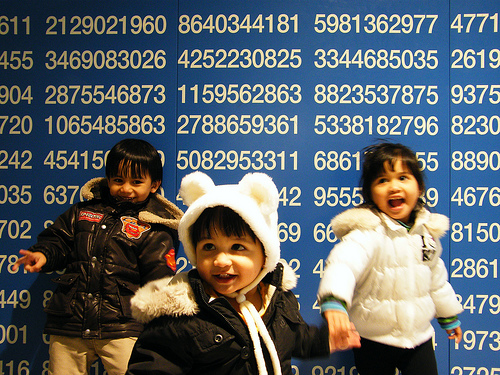# Lab 7.2 - Happy NumbersA happy number isn't really anything profound, just a little bit of recreational mathematics thought up by a mathematician's daughter. A happy number is any number that eventually reduces to 1 when the following process is used: take the sum of the squares of its digits, and continue iterating this process until it yields 1, or produces an infinite loop. For example, 7 is a happy number, as the sequence for 7 is:
```     7 ^ 2 = 49
4 ^ 2 + 9 ^ 2 = 16 + 81 = 97
9 ^ 2 + 7 ^ 2 = 81 + 49 = 130
1 ^ 2 + 3 ^ 2 + 0 ^ 2 = 1 + 9 + 0 = 10
1 ^ 2 + 0 ^ 2 = 1 + 0 = 1
```
Since the sequence ends with 1, it is a happy number. An unhappy number loops at some point in the series, as is the case with 4:
```     4 ^ 2 = 16
1 ^ 2 + 6 ^ 2 = 1 + 36 = 37
3 ^ 2  + 7 ^ 2 = 9 + 49 = 58
5 ^ 2 +8 ^ 2 = 25 + 64 = 89
8 ^ 2 + 9 ^ 2 = 64 + 81 = 145
1 ^ 2 + 4 ^ 2 + 5 ^ 2 = 1 + 16 + 25 = 42
4 ^ 2 + 2 ^ 2 = 16 + 4 = 20
2 ^ 2 + 0 ^ 2 = 4
```
... which loops us back to 4. Since it's an infinite loop, it is not a happy number.

Write a program that takes a numerical value from the user and determines whether or not it is a happy number. Use a dynamic array to keep a history of previous answers, and a linear search of that array to determine whether a loop has started.

This is another lab you're going to want to design in your notes first. On this one it's optional, but if you're having problems that could have been solved by designing ahead of time, you'll probably never hear the end of it.

Here's sample data and answers that you should get:
Input Result
1 Happy
3 Unhappy
23 Happy
42 Unhappy
9001 Happy

HINTS: Your program shouldn't crash, even if the user is dumb and doesn't put in a number. I'd give you a hint about isolating individual digits in an answer, but I wouldn't want to String you along.

Design Tip: There's only two different possible results (happy and unhappy), so it's possible that two different numbers give the same result, but the program appears to not do anything when you hit the button. So make it so that when the user starts typing a new number, the result becomes blank, like it was at the start of the program.

ToDo List:
• Program uses a dynamic array.
• Each subsequent iteration of the happy number algorithm uses a sequential search to find out if the current iteration value is a repeat of an earlier value.
• Demonstrates good visual design and software engineering practices.
• Check the program with the instructor.
• All other steps are complete, and the lab is turned in.